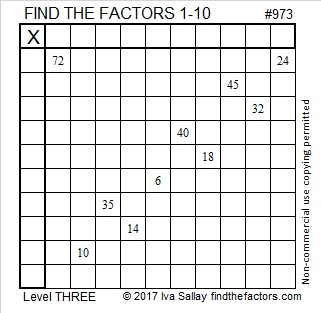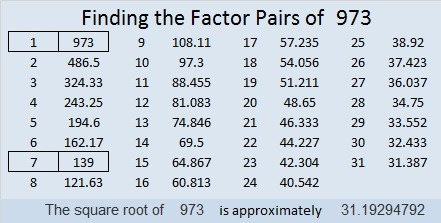973 and Level 3

If this were a Find the Factors 1 – 12 puzzle, then clues 72 and 24 would have three different possible common factors, but that isn’t the kind of puzzle it is. It is just a Find the Factors 1 – 10 puzzle, and there is just one possible common factor that will put only factors from 1 – 10 in the first column and the top row. Do you know that common factor? If you do, you will be well on your way to solving the puzzle!Print the puzzles or type the solution in this excel file: 10-factors-968-977

Here are a few facts about the number 973:

It is palindrome 191 in BASE 27 because 1(27²) + 9(27) + 1(1) = 973

• 973 is a composite number.
• Prime factorization: 973 = 7 × 139
• The exponents in the prime factorization are 1 and 1. Adding one to each and multiplying we get (1 + 1)(1 + 1) = 2 × 2 = 4. Therefore 973 has exactly 4 factors.
• Factors of 973: 1, 7, 139, 973
• Factor pairs: 973 = 1 × 973 or 7 × 139
• 973 has no square factors that allow its square root to be simplified. √973 ≈ 31.1929